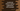# JavaScript filter method explanation with example## How to use filter() method in JavaScript array to create a new array:

The filter() method creates a new array by using a different function. It takes one function as the parameter, uses it as the predicate and returns one new array holding all the elements those passed the function.

In this post, we will learn how to use filter method with different examples.

### Syntax of filter:

We can use this method with an arrow function, callback function or with an inline callback function.

#### Arrow function:

It is defined as like below:

``filter((e, i, arr) => {}, thisArg);``

The arrow function is passed as a parameter to the filter method. Here,

• e is the element currently being processed in the array.
• i is an optional value. It is the index of the currently being processed element of the array.
• arr is also an optional value. It is the original array.
• thisArg is an optional value and it can be used as this while executing the callback function.

#### Callback function:

With callback function, it looks as like below:

``filter(callbackFunction, thisArg);``

The first parameter callbackFunction is the callback function that is used to find the filtered data. thisArg is optional.

#### Inline callback function:

We can also use an inline callback function.

``filter(function(e, i, arr) {}, thisArg);``

This is similar to the definition of the arrow function. The definition is similar to the arrow function definition.

### Return value of filter():

filter() method calls the argument function on each element of the array. It creates a new array by finding all values for which the argument function returns true. For other elements, for which it returns false, are skipped.

Let’s learn how filter() works with different examples.

### Example of filter() with callback function:

Let’s take an example of filter() with a callback function. The below program finds all even numbers from an array:

``````function isEven(e) {
return e % 2 === 0;
}

let givenArray = [1, 2, 3, 4, 5, 6, 7, 8, 9, 10];

console.log("givenArray: ", givenArray);

let evenValues = givenArray.filter(isEven);

console.log("evenValues: ", evenValues);``````

It prints:

``````givenArray:  [
1, 2, 3, 4,  5,
6, 7, 8, 9, 10
]
evenValues:  [ 2, 4, 6, 8, 10 ]``````

givenArray is the original array. We are using filter to find all even values in this array and this data is stored in evenValues.

### Example of filter() with inline callback function:

Let’s try filter with an inline callback function. We will use the same example:

``````let givenArray = [1, 2, 3, 4, 5, 6, 7, 8, 9, 10];

console.log("givenArray: ", givenArray);

let evenValues = givenArray.filter(function isEven(e) {
return e % 2 === 0;
});

console.log("evenValues: ", evenValues);``````

It will give the same output.

### Example of filter() with arrow function:

Let’s try filter() with an arrow function:

``````let givenArray = [1, 2, 3, 4, 5, 6, 7, 8, 9, 10];

console.log("givenArray: ", givenArray);

let evenValues = givenArray.filter(e => e % 2 === 0);

console.log("evenValues: ", evenValues);``````

The output will be same.

### Example with an array of objects:

Let’s try with an array of objects.

``````let users = [
{
id: 1,
name: "Alex",
},
{
id: 2,
name: "Bob",
},
{
name: "Chandler",
},
{
id: 3,
name: "Daisy",
},
{
name: "Ela",
},
];

console.log("users: ", users);

let validUsers = users.filter((e) => e?.id);

console.log("validUsers: ", validUsers);``````

In this example, an array of objects is given. We are using filter to filter out all valid objects in the array. An object is called valid if it has an id.

If you run this program, it will print the below output:

``````users:  [
{ id: 1, name: 'Alex' },
{ id: 2, name: 'Bob' },
{ name: 'Chandler' },
{ id: 3, name: 'Daisy' },
{ name: 'Ela' }
]
validUsers:  [
{ id: 1, name: 'Alex' },
{ id: 2, name: 'Bob' },
{ id: 3, name: 'Daisy' }
]``````